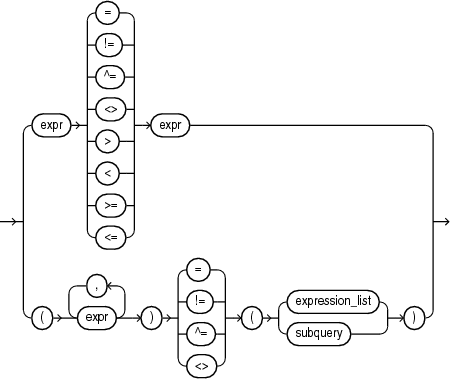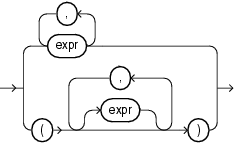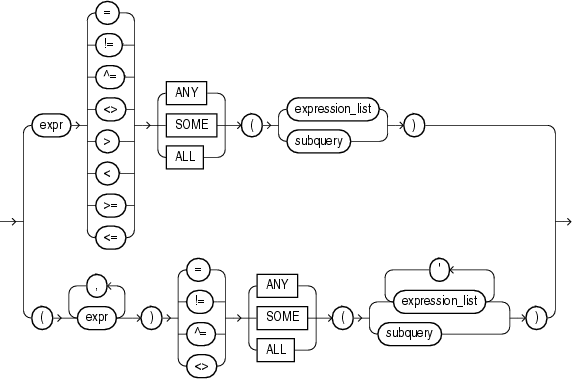## Comparison Conditions

Comparison conditions compare one expression with another. The result of such a comparison can be `TRUE`, `FALSE`, or `UNKNOWN`.

Large objects (LOBs) are not supported in comparison conditions. However, you can use PL/SQL programs for comparisons on `CLOB` data.

When comparing numeric expressions, Oracle uses numeric precedence to determine whether the condition compares `NUMBER`, `BINARY_FLOAT`, or `BINARY_DOUBLE` values. Refer to Numeric Precedence for information on numeric precedence.

When comparing character expressions, Oracle uses the rules described in Data Type Comparison Rules. The rules define how the character sets of the expressions are aligned before the comparison, the use of binary or linguistic comparison (collation), the use of blank-padded comparison semantics, and the restrictions resulting from limits imposed on collation keys, including reporting of the error `ORA-12742:` `unable` `to` `create` `the` `collation` `key`.

Two objects of nonscalar type are comparable if they are of the same named type and there is a one-to-one correspondence between their elements. In addition, nested tables of user-defined object types, even if their elements are comparable, must have `MAP` methods defined on them to be used in equality or `IN` conditions.

See Also:

Oracle Database Object-Relational Developer's Guide for information on using `MAP` methods to compare objects

Table 6-2 lists comparison conditions.

Table 6-2 Comparison Conditions

Type of Condition Purpose Example

`=`

Equality test.

```SELECT *
FROM employees
WHERE salary = 2500
ORDER BY employee_id;```

`!=`

`^=`

`<>`

Inequality test.

```SELECT *
FROM employees
WHERE salary != 2500
ORDER BY employee_id;```

`>`

`<`

Greater-than and less-than tests.

```SELECT * FROM employees
WHERE salary > 2500
ORDER BY employee_id;
SELECT * FROM employees
WHERE salary < 2500
ORDER BY employee_id;```

`>=`

`<=`

Greater-than-or-equal-to and less-than-or-equal-to tests.

```SELECT * FROM employees
WHERE salary >= 2500
ORDER BY employee_id;
SELECT * FROM employees
WHERE salary <= 2500
ORDER BY employee_id;```

`op ANY`

`op SOME`

"op" must be one of =, !=, >, <, <=, or >=.

`op ANY` compares a value on the left side either to each value in a list, or to each value returned by a query, whichever is specified on the right side, using the condition `op`.

If any of these comparisons returns TRUE, `op ANY` returns `TRUE`.

If all of these comparisons return `FALSE`, or the subquery on the right side returns no rows, `op ANY` returns `FALSE`. Otherwise, the return value is `UNKNOWN`.

`op ANY` and `op SOME` are synonymous.

```SELECT * FROM employees
WHERE salary = ANY
(SELECT salary
FROM employees
WHERE department_id = 30)
ORDER BY employee_id;```

` op ALL`

"op" must be one of =, !=, >, <, <=, or >=.

`op ALL` compares a value on the left side either to each value in a list, or to each value returned by a subquery, whichever is specified on the right side, using the condition `op`.

If any of these comparisons returns `FALSE`,` op ANY` returns `FALSE`.

If all of these comparisons return `TRUE`, or the subquery on the right side returns no rows, `op ALL` returns `TRUE` . Otherwise, the return value is `UNKNOWN`.

```SELECT * FROM employees
WHERE salary >=
ALL (1400, 3000)
ORDER BY employee_id;```

### Simple Comparison Conditions

A simple comparison condition specifies a comparison with expressions or subquery results.

simple_comparison_condition::=Description of the illustration simple_comparison_condition.eps

expression_list::=Description of the illustration expression_list.eps

If you use the lower form of this condition with a single expression to the left of the operator, then you can use the upper or lower form of `expression_list`. If you use the lower form of this condition with multiple expressions to the left of the operator, then you must use the lower form of `expression_list`. In either case, the expressions in `expression_list` must match in number and data type the expressions to the left of the operator. If you specify `subquery`, then the values returned by the subquery must match in number and data type the expressions to the left of the operator.

See Also:

Expression Lists for more information about combining expressions and SELECT for information about subqueries

### Group Comparison Conditions

A group comparison condition specifies a comparison with any or all members in a list or subquery.

group_comparison_condition::=Description of the illustration group_comparison_condition.eps

expression_list::=Description of the illustration expression_list.eps

If you use the upper form of this condition (with a single expression to the left of the operator), then you must use the upper form of `expression_list`. If you use the lower form of this condition (with multiple expressions to the left of the operator), then you must use the lower form of `expression_list`, and the expressions in each `expression_list` must match in number and data type the expressions to the left of the operator. If you specify `subquery`, then the values returned by the subquery must match in number and data type the expressions to the left of the operator.

See Also: